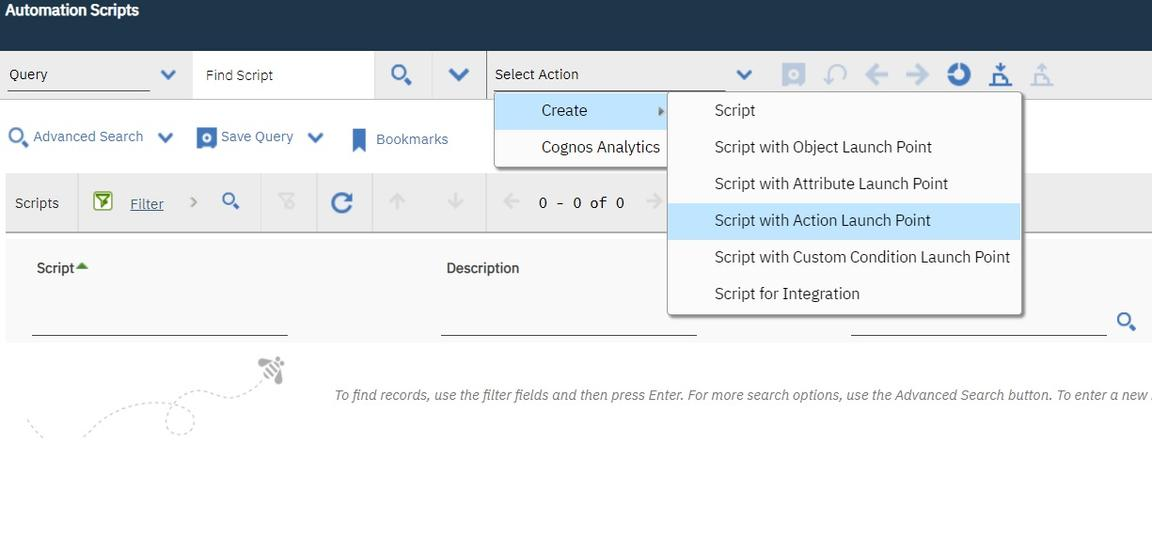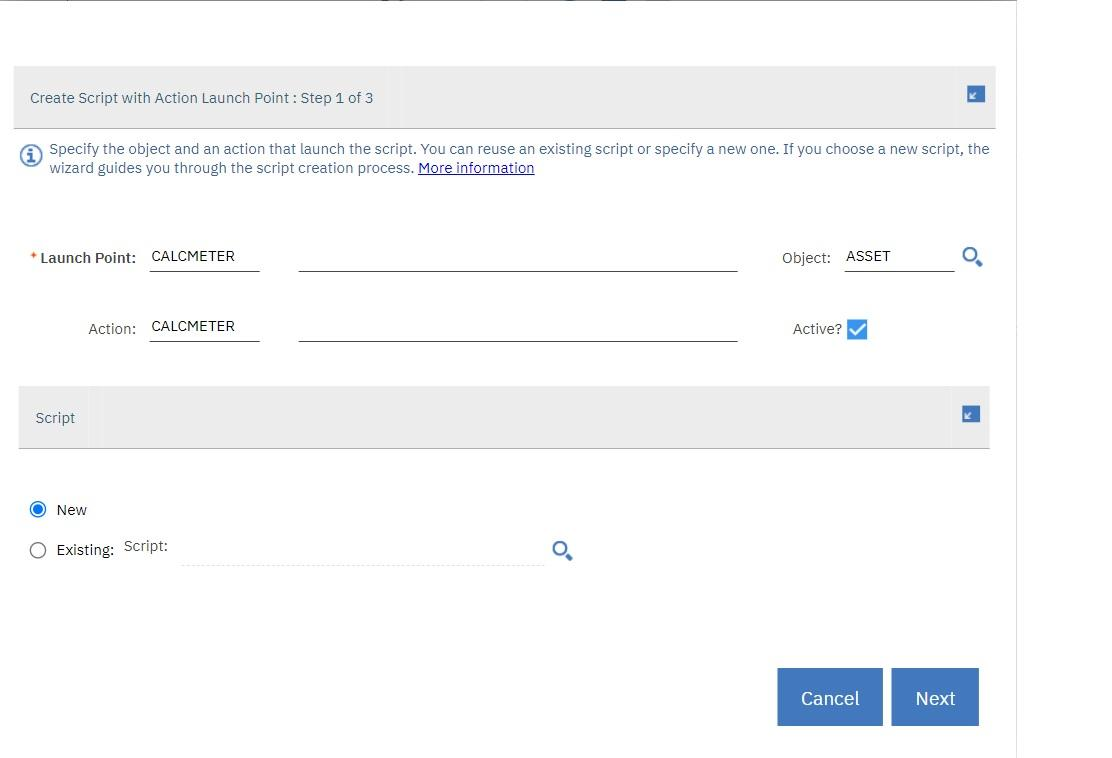# Action launch point

## Action launch point

The Maximo Actions framework in Maximo has a built in library of Actions which can be invoked from Workflows, Escalations, UI Menus, UI Buttons and many other components. More often than not those built in library of Actions are not enough and implementers have to develop their own custom Actions in JAVA only. Scripting addresses this concern where an Action can be scripted with a scripting language of your choice using Jython and JavaScript. You can use a scripted Action to do some calculated meters for Assets. The following screenshot shows how to launch the Action Launch Point wizard.The requirement is to be able to calculate an Asset meter value based on some other meters associated with that Asset. For example, you want to calculate the value of the PRESSURE meter, based on the IN-PRESSUR and O-PRESSUR meter such that the last reading of the PRESSURE meter is the summation of the IN and O-PRESSUR meters readings. Assume that you choose to do it offline as opposed to in real time, for which you would have to use Object Launch points to trap meter modification events. The simplest way to do offline actions repeatedly in Maximo is to use Escalations, which are nothing but cron jobs that execute a predefined Action in the context of an MBO. Instead of writing the Action java code you will use a script as shown in the following steps:

1. Define a relationship called assetmeterip [asset meter input pressure] using the DB-Config application, which relates an Asset to the Asset meter named IN-PRESSUR. Use the following where clause:
`assetnum=:assetnum and siteid=:siteid and metername='IN-PRESSUR'`

Similarly, define the other two relationships:

Realtion nameRelation where
assetmeteropassetnum=:assetnum and siteid=:siteid and metername=‘O-PRESSUR’
assetmeterpassetnum=:assetnum and siteid=:siteid and metername=‘PRESSURE’
1. Use the Action Launch Point wizard to define the Action Launch Point.Though this wizard will create the Action behind the scene, it is the responsibility of the implementer to attach that Action to the escalation, workflow or the UI button/menu. By default, the launch point name is used as the name of the Action, but you can modify the value to suit your naming convention. However, it is better to avoid renaming it because of name confusion and conflicts later on. In the first step of the wizard you saw that the object name is optional, which is in-line with the Maximo Action framework where an Action may or may not be associated with a Maximo object. In this case however, you want to specify the object as Asset as the Action is specific to the Asset MBO. Since you are defining a new script you must choose the New Script option.

The next page is the bindings page where you have to define the variables that you will use for the script and the variable bindings. You require the last reading value of the IN-PRESSUR and O-PRESSUR meters and must set the calculated value to the new reading attribute of the PRESSURE meter. However, you do not want to set the value if the calculated value is the same as the last reading value of the PRESSURE meter because that would generate meter reading history even though the reading never got modified. To check this, you would need the last reading value of the PRESSURE meter. So the following variable bindings will look like:

Variable nameVariable typeBinding
`y=float(iplr)+float(olr)if y!=float(plr):  pnr=str(y)`
The `if` check in the second line takes care of not updating the `pnr` value if the calculated value is the same as the `plr` [PRESSURE meters last reading]. This calculation was implemented as an example. In real implementations it can be any complicated mathematical calculation as per your business requirement, only limited by the mathematical support provided by the scripting language of your choice.
`exists (select assetnum from assetmeter where metername='IN-PRESSUR' andassetnum=asset.assetnum and siteid=asset.siteid) and exists (select assetnum from assetmeterwhere metername='O-PRESSUR' and assetnum=asset.assetnum and siteid=asset.siteid) andexists (select assetnum from assetmeter where metername='PRESSURE' andassetnum=asset.assetnum and siteid=asset.siteid)`